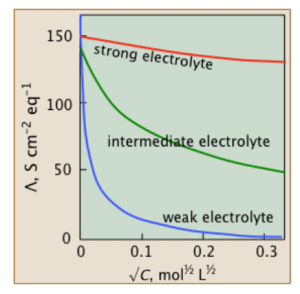Courses

# Variation of Conductivity and Molar Conductivity Class 12 Notes | EduRev

## Chemistry Class 12

Created by: Mohit Rajpoot

## Class 12 : Variation of Conductivity and Molar Conductivity Class 12 Notes | EduRev

The document Variation of Conductivity and Molar Conductivity Class 12 Notes | EduRev is a part of the Class 12 Course Chemistry Class 12.
All you need of Class 12 at this link: Class 12

What is Specific Conductivity?
Specific conductivity or conductivity of an electrolytic solution at any given concentration is the conductance of unit volume of solution. It is the conductance when kept between two platinum electrodes with a unit area of cross-section.
The electrodes are at a distance of unit length.
Conductivity decreases with a decrease in concentration as the number of ions per unit volume that carry the current in a solution decrease on dilution.
The molar conductivity of a solution at a given concentration is the conductance of volume V of the solution containing one mole of electrolyte kept between two electrodes with an area of cross section A and distance of unit length.

Ʌm = К/c

Here, c = concentration in moles per volume, К = specific conductivity and Ʌm = molar conductivity. As the solution contains only one mole of electrolyte, the above equation can be modified as:

Ʌm = КV

Change in Molar Conductivity:

Molar conductivity increases with a decrease in concentration. This happens because the total volume, V, of the solution containing one mole of electrolyte also increases. Upon dilution, the concentration decreases.
Furthermore, when the concentration approaches zero, the molar conductivity of the solution is known as limiting molar conductivity, Ë°m. Variation of molar conductivity with concentration is different for both, strong and weak electrolytes.Fig: Variation of molar conductivity with concentrationVariation of Molar Conductivity with Concentration of Strong Electrolytes:
For strong electrolytes, the molar conductivity increases slowly with the dilution. The plot of the molar conductivity and c1/2 is a straight line having y-intercept equal to Ë°m. The value of limiting molar conductivity, Ë°m can be determined from the graph or with the help of Kohlrausch law.

For strong electrolytes, the molar conductivity increases slowly with the dilution. Thus, the plot of the molar conductivity and c1/2 is a straight line having y-intercept equal to Ë°m. The general equation for the plot is:

Ʌm = Ë°m − Ac1/2
Where −A is a constant equal to the slope of the line. Furthermore, the value of “A” for a given solvent depends on the type of electrolyte at a particular temperature. Hence, it differs from solution to solution.

Variation of Molar Conductivity with Concentration for Weak Electrolytes:

For weak electrolytes, the graph plotted between molar conductivity and c1/2 (where c is the concentration) is not a straight line. This is because weak electrolytes have lower molar conductivities and lower degree of dissociation at higher concentrations which increases steeply at lower concentrations. Hence, we use Kohlrausch law of independent migration of ions for determining to limit molar conductivity, Ëm° of weak electrolytes.

Solved Example
Ques: How does concentration of a solution affect its specific conductivity?
Ans:
Specific Conductivity decreases with a decrease in concentration. Since the number of ions per unit volume that carry current in a solution decrease on dilution. Hence, concentration and conductivity are directly proportional to each other.

Offer running on EduRev: Apply code STAYHOME200 to get INR 200 off on our premium plan EduRev Infinity!

,

,

,

,

,

,

,

,

,

,

,

,

,

,

,

,

,

,

,

,

,

;